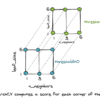# Search results for "MinMaxScaler"## How to Use StandardScaler and MinMaxScaler Transforms in Python

Many machine learning algorithms perform better when numerical input variables are scaled to a standard range. This includes algorithms that use a weighted sum of the input, like linear regression, and algorithms that use distance measures, like k-nearest neighbors. The two most popular techniques for scaling numerical data prior to modeling are normalization and standardization. […]## Understanding Simple Recurrent Neural Networks In Keras

This tutorial is designed for anyone looking for an understanding of how recurrent neural networks (RNN) work and how to use them via the Keras deep learning library. While all the methods required for solving problems and building applications are provided by the Keras library, it is also important to gain an insight on how […]## Adding A Custom Attention Layer To Recurrent Neural Network In Keras

Deep learning networks have gained immense popularity in the past few years. The ‘attention mechanism’ is integrated with the deep learning networks to improve their performance. Adding attention component to the network has shown significant improvement in tasks such as machine translation, image recognition, text summarization and similar applications. This tutorial shows how to add […]## How to Make Predictions with Keras

Once you choose and fit a final deep learning model in Keras, you can use it to make predictions on new data instances. There is some confusion amongst beginners about how exactly to do this. I often see questions such as: How do I make predictions with my model in Keras? In this tutorial, you […]## Easier Experimenting in Python

When we work on a machine learning project, we quite often need to experiment with multiple alternatives. Some features in Python allow us to try out different options without much effort. In this tutorial, we are going to see some tips to make our experiments faster. After finishing this tutorial, you will learn: How to […]## Modeling Pipeline Optimization With scikit-learn

This tutorial presents two essential concepts in data science and automated learning. One is the machine learning pipeline, and the second is its optimization. These two principles are the key to implementing any successful intelligent system based on machine learning. A machine learning pipeline can be created by putting together a sequence of steps involved […]## Prediction Intervals for Deep Learning Neural Networks

Prediction intervals provide a measure of uncertainty for predictions on regression problems. For example, a 95% prediction interval indicates that 95 out of 100 times, the true value will fall between the lower and upper values of the range. This is different from a simple point prediction that might represent the center of the uncertainty […]## How to Develop a Neural Net for Predicting Car Insurance Payout

Developing a neural network predictive model for a new dataset can be challenging. One approach is to first inspect the dataset and develop ideas for what models might work, then explore the learning dynamics of simple models on the dataset, then finally develop and tune a model for the dataset with a robust test harness. […]## Autoencoder Feature Extraction for Regression

Autoencoder is a type of neural network that can be used to learn a compressed representation of raw data. An autoencoder is composed of encoder and a decoder sub-models. The encoder compresses the input and the decoder attempts to recreate the input from the compressed version provided by the encoder. After training, the encoder model […]## Autoencoder Feature Extraction for Classification

Autoencoder is a type of neural network that can be used to learn a compressed representation of raw data. An autoencoder is composed of an encoder and a decoder sub-models. The encoder compresses the input and the decoder attempts to recreate the input from the compressed version provided by the encoder. After training, the encoder […]